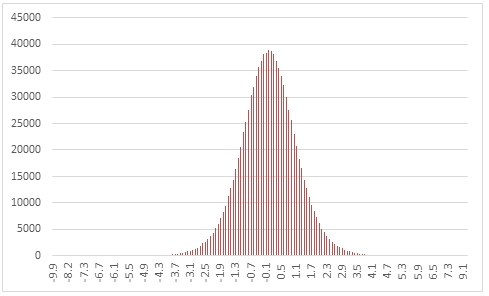# SQL Server random t-distribution

RANDTDIST

Updated: 31 March 2014

Use the table-valued function RANDTDIST to generate a sequence of random numbers from Student's t distribution with parameter @df.
Syntax
SELECT * FROM [wctMath].[wct].[RANDTDIST](
<@Rows, int,>
,<@df, float,>)
Arguments
@Rows
the number of rows to generate. @Rows must be of the type int or of a type that implicitly converts to int.
@df
the degrees of freedom. @df must be of the type float or of a type that implicitly converts to float.
Return Types
RETURNS TABLE (
[Seq] [int] NULL,
[X] [float] NULL
)
Remarks
·         @df must be greater than zero.
·         If @df is NULL then @Shape is set to 1.
·         If @Rows is less than 1 then no rows are returned.
Examples
In this example we create a sequence 1,000,000 random numbers rounded to one decimal place from a Student's t distribution with @df = 10, COUNT the results, paste the results into Excel and graph them.
SELECT
X,
COUNT(*) as [COUNT]
FROM (
SELECT
ROUND(X,1) as X
FROM
wct.RANDTDIST(
1000000, --@Rows
10       --@df
)
)n
GROUP BY
X
ORDER BY
X
This produces the following result.In this example we generate 1,000,000 random numbers from a t distribution with @lambda of 6. We calculate the mean, standard deviation, skewness, and excess kurtosis from the resultant table and compare those values to the expected values for the distribution.
DECLARE @size as int = 1000000
DECLARE @lambda as float = 6
DECLARE @mean as float = 0
DECLARE @var as float =
CASE
WHEN @lambda <= 2 THEN NULL
ELSE @lambda /(@lambda -2)
END
DECLARE @stdev as float = SQRT(@var)
DECLARE @skew as float = 0
DECLARE @kurt as float =
CASE
WHEN @lambda <= 4 THEN NULL
ELSE 6/(@lambda -4)
END

SELECT
stat,
[RANDTDIST],
[EXPECTED]
FROM (
SELECT
x.*
FROM (
SELECT
AVG(x) as mean_TDIST,
STDEVP(x) as stdev_TDIST,
wct.SKEWNESS_P(x) as skew_TDIST,
wct.KURTOSIS_P(x) as kurt_TDIST
FROM
wct.RANDTDIST(@size, @lambda)
)n
CROSS APPLY(
VALUES
('RANDTDIST','avg', mean_TDIST),
('RANDTDIST','stdev', stdev_TDIST),
('RANDTDIST','skew', skew_TDIST),
('RANDTDIST','kurt', kurt_TDIST),
('EXPECTED','avg',@mean),
('EXPECTED','stdev',@stdev),
('EXPECTED','skew',@skew),
('EXPECTED','kurt',@kurt)
)x(fn_name,stat,val_stat)
)d
PIVOT(sum(val_stat) FOR fn_name in([RANDTDIST],[EXPECTED])) P

This produces the following result (your result will be different).

 stat RANDTDIST EXPECTED avg -0.003034901 0 kurt 3.090949021 3 skew 0.023943502 0 stdev 1.224957684 1.224744871

### SupportCopyright 2008-2023 Westclintech LLC         Privacy Policy        Terms of Service